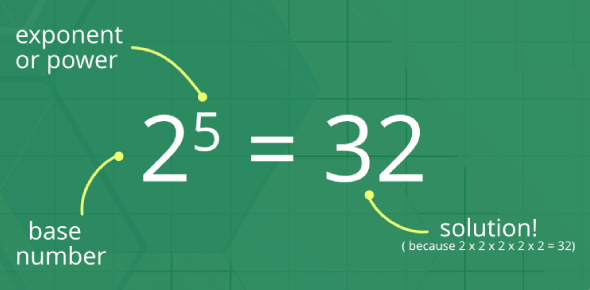# Ultimate Mathematics Test Questions! Quiz

10 Questions | Total Attempts: 56079SettingsAre you any good at mathematics? Do you imagine you can succeed at taking this quiz? Math is the science of structure and order, which has changed from elemental practices of counting, measuring, and describing the object. Some mathematics branches are characterized by a strict set of rules and parameters, with examples including algebra, geometry, and calculus. Taking this quiz will help you discover if you are a math whiz!

Questions and Answers
• 1.
Estimate the critical value of t for a 98% confidence interval with df = 20
• A.

2.528

• B.

2.518

• C.

1.325

• D.

2.539

• E.

2.330

• 2.
Find the P-value for |t| > 1.76 with 24 degrees of freedom.
• A.

.0911

• B.

.0456

• C.

.1562

• D.

.9544

• E.

.0781

• 3.
You want to estimate the average gas price in your city for a gallon of regular gas. From your sample of 15 gas stations, you calculate a 95% confidence interval of (\$1.98, \$2.16).  Interpret the confidence interval.
• A.

You are 95% sure that the average price for a gallon of gas in your city is between \$1.98 and \$2.16.

• B.

If you took many samples of gas stations in your city, about 95% of them would produce this confidence interval.

• C.

95% of all samples of gas stations will have average costs between \$1.98 and \$2.16.

• D.

You are 95% sure that gas stations in this sample have average costs between \$1.98 and \$2.16.

• E.

You are 95% confident that the average price for a gallon of gas in the country is within \$0.09 of \$2.07.

• 4.
A confidence interval estimate is determined from the GPAs of a simple random sample of n students.  All other things being equal, which of the following will result in a smaller margin of error?
• A.

A smaller confidence level

• B.

A larger sample standard deviation

• C.

A smaller sample size

• D.

A larger population size

• E.

A smaller sample mean

• 5.
In an SRS of 80 teenagers, the average number of texts handled in a day was 50 with a standard deviation of 15.  What is the 96% confidence interval for the average number of texts handled by teens daily?
• A.
• B.
• C.
• D.
• E.
• 6.
The number of accidents per day at a large factory is noted for each of 64 days with a mean of 3.58 and a standard deviation of 1.52.  With that degree of confidence, we can assert that the mean number of accidents per day at the factory is between 3.20 and 3.96.
• A.

95%

• B.

48%

• C.

63%

• D.

90%

• E.

99%

• 7.
Two confidence interval estimates are obtained:  I (28.5, 34.5) and II (30.3, 38.2).
1. If the sample sizes are the same, which has a larger standard deviation?
2. If the sample standard deviations are the same, which has a larger size?
• A.

1. I 2. II

• B.

1. I 2. I

• C.

1. II 2. I

• D.

1. II 2. II

• E.

More information is needed to answer these questions

• 8.
A company selling home appliances claims that the accompanying instruction manuals are written at a 6th-grade reading level.  An English teacher believes that the true figure is higher and with the help of an AP Stat student runs a hypothesis test.  The student randomly picks one page from each of 25 of the company's instructions manuals, and the teacher subjects the pages to a standard readability test.  The reading levels of the 25 pages are given in the following table:   Reading Grade Level 5 6 7 8 9 10 Number of pages 6 10 4 2 2 1 Is there statistical evidence to support the English teacher's belief?
• A.

No, because the p-value is greater than .10

• B.

Yes, the p-value is between .01 and .05 indicating some evidence for the teacher's belief.

• C.

Yes, the p-value is between .001 and .01 indicating strong evidence for the teacher's belief.

• D.

Yes, the p-value is between .05 and .10 indicating strong evidence for the teacher's belief.

• E.

Yes, the p-value is less than .001 indicating strong evidence for the teacher's belief.

• 9.
Thirty students volunteer to test which of two strategies for taking multiple-choice exams leads to higher average results.  Each student flips a coin, and if heads, uses Strategy A on the first exam and then Strategy B on the second exam, while if tails, uses Strategy B first and then Strategy A.  The average of all 30 Strategy A result is then compared to the average of all 30 Strategy B results.  What is the conclusion at the 5% significance level if a two-sample hypothesis test, , results in a p-value of .18?
• A.

The observed difference in average scores is significant.

• B.

The observed difference in average scores is not significant.

• C.

A conclusion is not possible without knowing the average scores resulting from using each strategy.

• D.

A conclusion is not possible without knowing the averages scores and the standard deviations resulting from using each strategy.

• E.

A two-sample hypothesis test should not be used here.

• 10.
Based on data from two very large independent samples, two students tested a hypothesis about equality of population means using α = 0.02. One student used a one-tail test and rejected the null hypothesis, but the other used a two-tail test and failed to reject the null. Which of these might have been their calculated value of t?
• A.

1.55

• B.

1.22

• C.

2.22

• D.

1.88

• E.

2.66

Related Topics